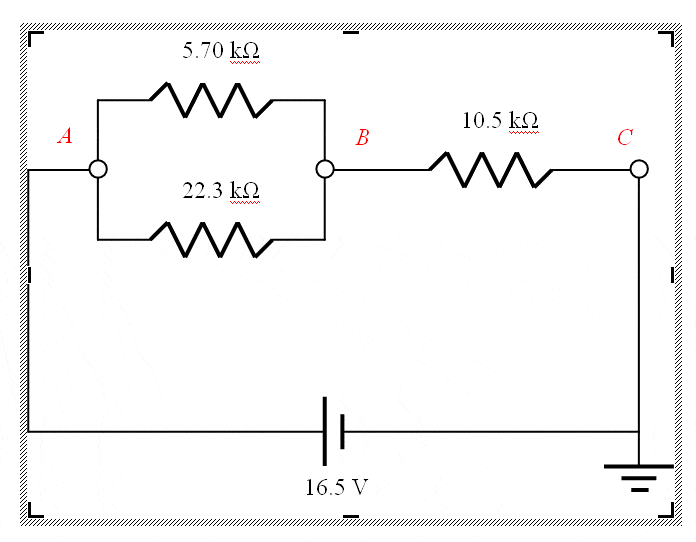# Undersanding Series and parallel resistors

In order to better understand of the project, I checked some resources of what is the difference between the Resistor in Series and in parallel.In the circuit shown, find the current in each resistor and the values of the potential at points A, B, and C.First I computed the combined resistance of the parallel resistors and got 4.54 kOhms. Then I added this to 10.5 to get 15.04 kOhms total resistance. So from Ohm’s law, the total current is I=V/R = 16.5 / 15040 = 1.0971 x 10-3 A. I get potentials at A, B, and C of 16.5, 11.5, and 0 volts. I think this is correct so far. To find the current through each resistor: For the 10.5 kOhm resistor, since it is in series, it makes sense to me that the current through this resistor must be equal to the total current: 1.0971 x 10-3 A. And that the current running through the parallel pair must also be 1.0971 x 10-3 A. But the voltage across this resistor is 16.5-11.5, or 5v. Using Ohm’s law, I=V/R, I get 5/10500 = 0.000476 A Which way is correct??? Thanks

The voltage drop between B and C is 11.52 V not 5 V, hence you need to calculate 11.52V / 10500 Ohm which is the expected 1.097 mA.

The 4.98V are dropped from A to B and consequently the the current divides into 0.874 mA in the top branch and 0.223 mA in the bottom branch adding up again to 1.097mA.

1 Like

Hi there. Are you using Particle products in your project? Please let us know.